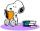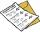# Star equation

Write digits instead of stars so that the sum of the written digits is odd and is true equality:

42 · ∗8 = 2 ∗∗∗

Result

b1 =  48
b2 =  68
c1 =  2016
c2 =  2856

#### Solution:Leave us a comment of example and its solution (i.e. if it is still somewhat unclear...):

Showing 0 comments:Be the first to comment!#### To solve this example are needed these knowledge from mathematics:

Do you have a linear equation or system of equations and looking for its solution? Or do you have quadratic equation?

## Next similar examples:

1. Number unknownAdela thought the two-digit number, she added it to its ten times and got 407. What number does she think?
2. Forest nurseryIn the forest nursery after winter, they found that 1/10 stems died out of them. For them, they land 193 new spruces. How many spruces are in the forest nursery?
3. I thinkI think a number. When I multiply it by five, and after that I subtract 477, I get the same number as if I multiplied it twice. What number do I think?
4. Apples 3Julka has 5 apples more than Hugo and four apples less than Annie. Hugo has 17 apples. How many apples has Julka and how Annie?
5. Eq1Solve equation: 4(a-3)=3(2a-5)
6. PopsiclesFrancis went to buy ice lollies. If he buy 8 popsicles he missed 4 USD. When he buy 7 popsicles, got back 1 USD. How many USD was a popsicle?
7. Seven timesWhich number seven times is just as higher as 27, how much is smaller than 29?
8. If-then equationIf 5x - 17 = -x + 7, then x =
9. Equation algebraogramSolve the equation: oco + ivo = cita How much has the task of solutions?
10. StudentsThe class has 22 students. 2 of which has assesement of 2. Count of assesement of 1 is three times more than count of students with assesement of 3. How many students has assesement of 1 and how many students has assesement of 1.
11. Roman numeralsWrite numbers written in Roman numerals as decimal.
12. Write decimalsWrite in the decimal system the short and advanced form of these numbers: a) four thousand seventy-nine b) five hundred and one thousand six hundred and ten c) nine million twenty-six
13. Addition of Roman numbersAdded together and write as decimal number: LXVII + MLXIV
14. CagesHonza had three cages (black, silver, gold) and three animals (guinea pig, rat and puppy). There was one animal in each cage. The golden cage stood to the left of the black cage. The silver cage stood on the right of the guinea pig cage. The rat was in the
15. ProductResult of the product of the numbers 1, 2, 3, 1, 2, 0 is:
16. Unknown number 16My number's tens is three times more then ones My number's ones is twice the number of thousands and my number's hundreds is half the number of of tens. I have two ones. which number am I?
17. Foot in busIt was 102 people on the bus. 28 girls had two dogs. A 11 girls had one dog. At the next stop seceded 5 dogs (even with their owners). They got two boys together with three dogs. The bus drove one driver. How many foot were in bus?